# Units - math word problems

1. Motor physics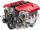Car traveling at 130 km/h on the highway. Pulling power of the motor is 8 kN. Determine engine power in kW.
2. Hurry - rush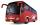At an average speed 7 km/h I will come from the school to the bus stop for 30 minutes. How fast I need to go if I need to get to the bus stop in 21 minutes?
3. AnglesThe triangle is one outer angle 158°54' and one internal angle 148°. Calculate the other internal angles of a triangle.
4. Price of state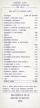Estimate the cost of the state based on following reason. State price is expressed as a percentage respectively share in the redistribution of national GDP. Employee creates monthly value € 868 (super-gross wage).Then tax him 35.2% employer contributions
5. Liters in cylinderDetermine the height at which level 24 liters of water in a cylindrical container having a bottom diameter 36 cm.
6. Chase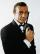Paul travel at 60 km/h. After 18 km ride found that he forgot an important book. His brother Thomas behind him carrying it. How fast must Thomas go to catch up with Paul in 31 minutes?
7. Pumps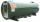6 pump fills the tank for 3 and a half days. How long will fill the tank 7 equally powerful pumps?
8. Complete constructionConstruct triangle ABC if hypotenuse c = 7 cm and angle ABC = 30 degrees. / Use Thales' theorem - circle /. Measure and write down the length of legs.
9. Sphere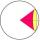Intersect between plane and a sphere is a circle with a radius of 60 mm. Cone whose base is this circle and whose apex is at the center of the sphere has a height of 34 mm. Calculate the surface area and volume of a sphere.
10. Sisters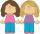Sisters Hanka and Vera goes mostly tograndmother by bicycle. Journey took Hanka 30 minutes and Vera 20 minutes. How long will Vera catch up with Hanka when started from home five minutes later than Hanka?
11. Clock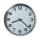How many hours are 15 days?
12. Cubic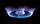Convert 660 m3 of natural gas volume to energy units kWh. Combustion of 1 cubic meter of natural gas is released energy 10.55 kWh.
13. Angles ratioIn a triangle ABC true relationship c is less than b and b is less than a. Internal angles of the triangle are in the ratio 5:4:9. The size of the internal angle beta is:
14. Vacation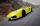Parents piggybacking children on vacation to its grandmother and grandfather at a city distant 150 kilometers. They agreed to meet halfway. Parents will be travel at 90 km/h, grandmother and grandfather at 60 km/h. Parents depart at 12:00 hours. When grand
15. Money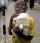Everyone complains about the lack of money. In fact, there is no shortage of money, but the relative scarcity of goods and things that people buy for money. Calculate what percentage of the price of all goods and services rose immediately, if everyone has
16. AbyssStone was pushed into the abyss: 2 seconds after we heard hitting the bottom. How deep is the abyss (neglecting air resistance)? (gravitational acceleration g = 9.81 m/s2 and the speed of sound in air v = 343 m/s)
17. Isosceles triangleWhat are the angles of an isosceles triangle ABC if its base is long a=5 m and has an arm b=4 m.
18. Car amortization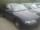Assume that the car has a life 13 years and new cost 24000 euros. Calculate how much a month is amortized cost of the car over its lifetime. Amortization is the gradual reduction of assets (cars, buildings), mainly due using or aging.
19. Racing track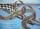On the racing track circling three cars. The first passes one circuit for 8 seconds, the second for 20 seconds and a third for 8 seconds. a) Calculate number of seconds since the start to catch all three cars together for the first time again across the s
20. Paper box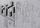Calculate the consumption of paper on the box-shaped quadrangular prism with rhombic footstall, base edge a=6 cm and the adjacent base edges forms an angle alpha = 60 °. Box height is 10 cm. How many m2 of the paper consumed 100 such boxes?

Do you have an interesting mathematical word problem that you can't solve it? Enter it, and we can try to solve it.

To this e-mail address, we will reply solution; solved examples are also published here. Please enter the e-mail correctly and check whether you don't have a full mailbox.

Please do not submit problems from current active competitions such as Mathematical Olympiad, correspondence seminars etc...